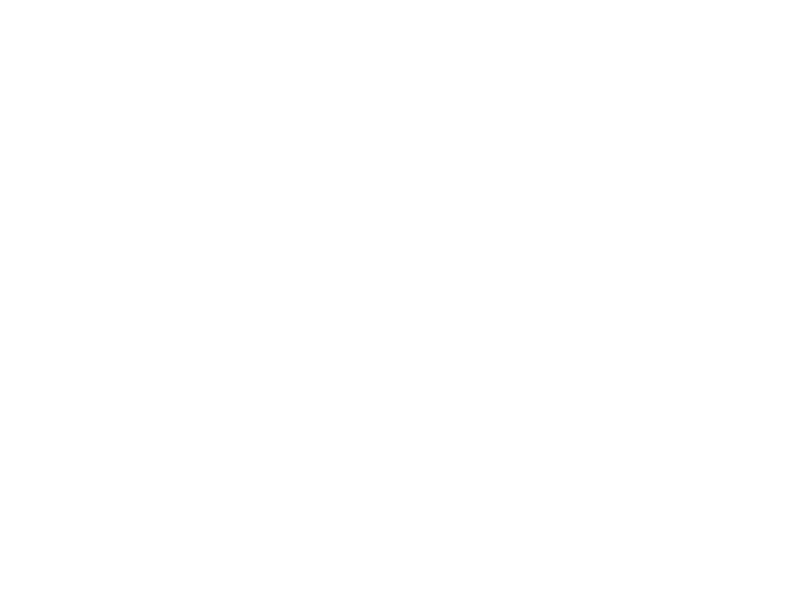# Patterns and Inductive Reasoning Inductive reasoning is reasoning

• Slides: 7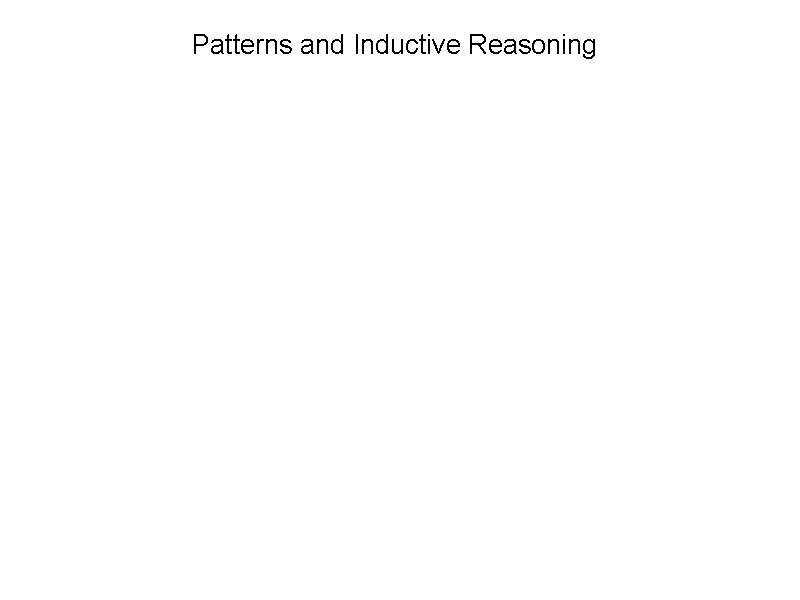Patterns and Inductive Reasoning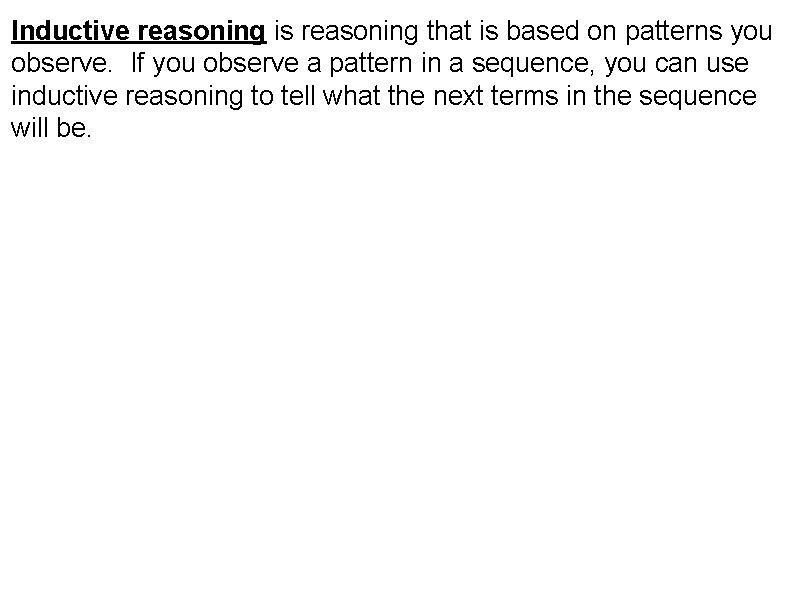Inductive reasoning is reasoning that is based on patterns you observe. If you observe a pattern in a sequence, you can use inductive reasoning to tell what the next terms in the sequence will be.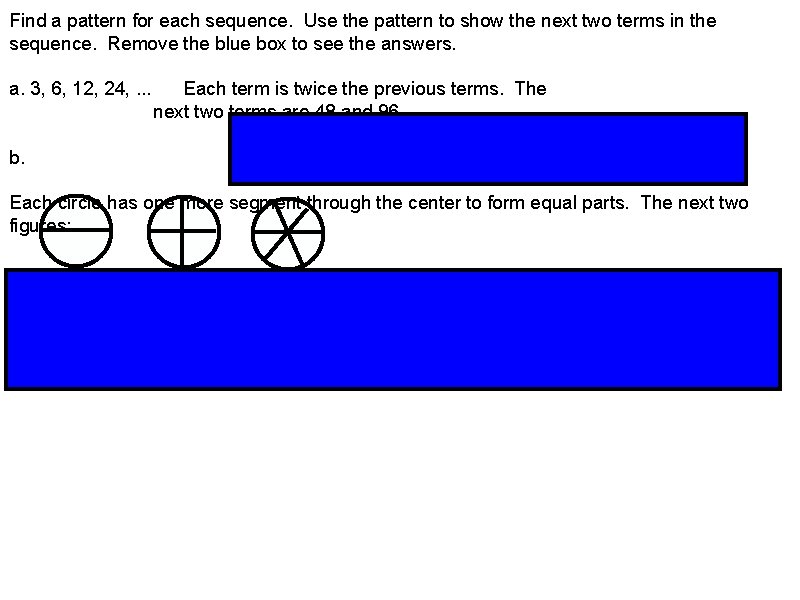Find a pattern for each sequence. Use the pattern to show the next two terms in the sequence. Remove the blue box to see the answers. a. 3, 6, 12, 24, . . . Each term is twice the previous terms. The next two terms are 48 and 96. b. Each circle has one more segment through the center to form equal parts. The next two figures: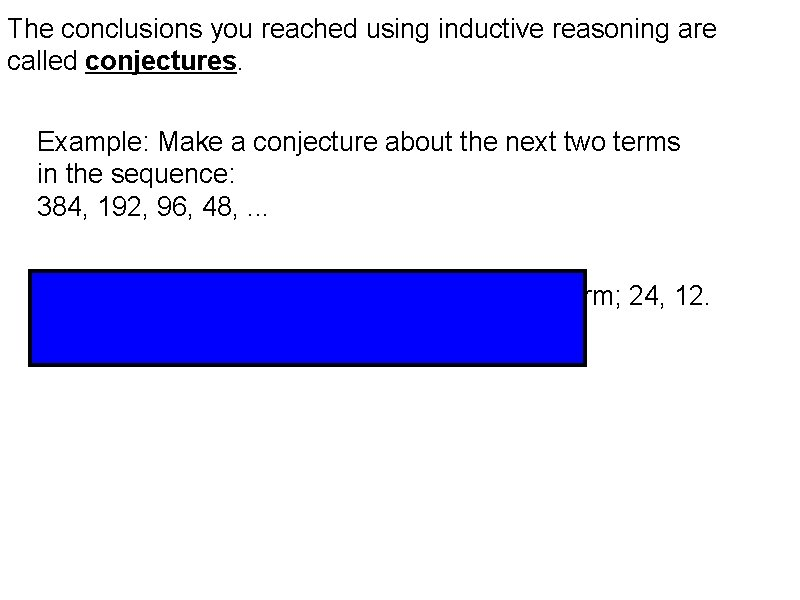The conclusions you reached using inductive reasoning are called conjectures. Example: Make a conjecture about the next two terms in the sequence: 384, 192, 96, 48, . . . Conjecture: Each term is half the preceding term; 24, 12.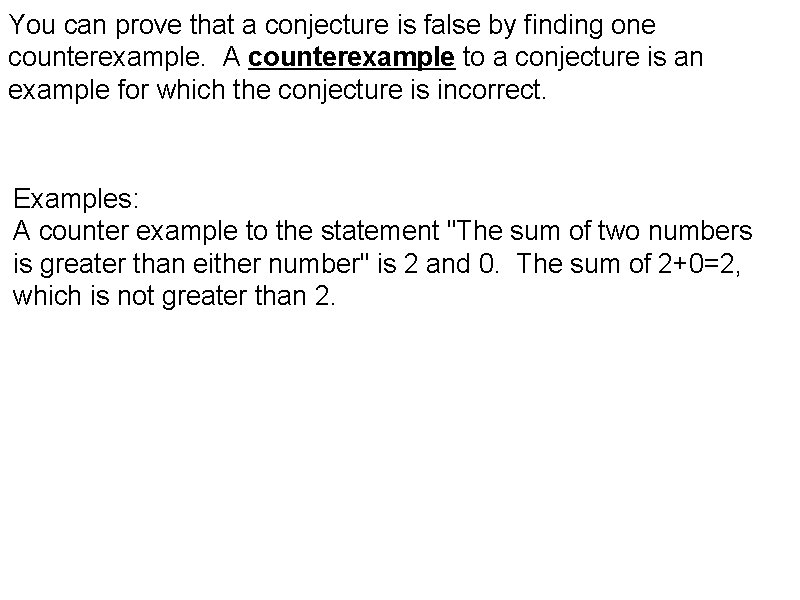You can prove that a conjecture is false by finding one counterexample. A counterexample to a conjecture is an example for which the conjecture is incorrect. Examples: A counter example to the statement "The sum of two numbers is greater than either number" is 2 and 0. The sum of 2+0=2, which is not greater than 2.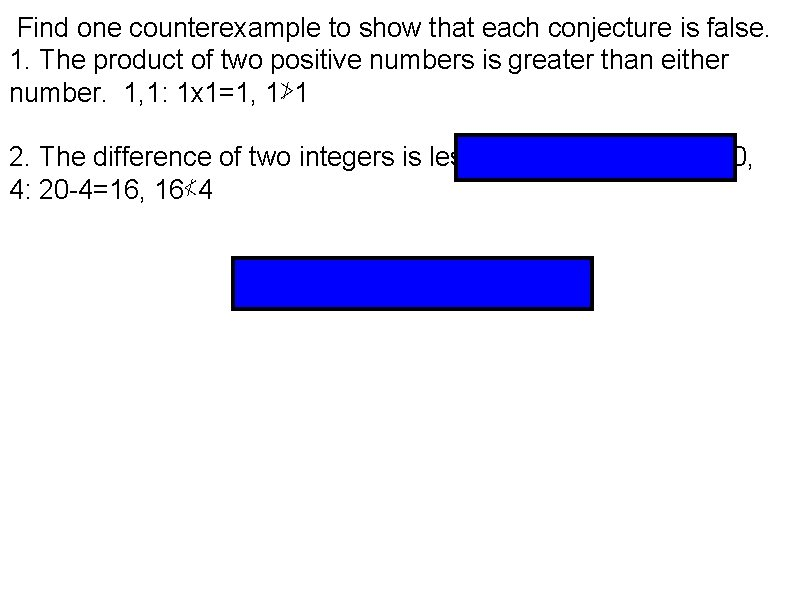Find one counterexample to show that each conjecture is false. 1. The product of two positive numbers is greater than either number. 1, 1: 1 x 1=1, 1≯ 1 2. The difference of two integers is less than either integer. 20, 4: 20 -4=16, 16≮ 4